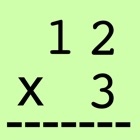Samurai Math

• SEARCH TYPE

All iPhone applications categories

All iPhone games categories# Samurai Math

by: 0 0

## Screenshots

Description

Just calculate 10 questions faster without making mistakes.

You can use the way to calculate on paper (step way) or mental arithmetic calculation (direct way).

Step mode (Input from right) is the way just like doing calculation on paper.
Direct mode (Input from left) is the way to input answer directly.

If you want to input faster, normally direct mode is faster.

But, the step mode is the basic of calculation, so if you are not confident with the way to calculate on paer (step way), then practice by step way first.

When you push Start button, game will start.
Step mode is default setting. You may change the mode by Dir(direct) button.

If your answer is correct at the first try, then you get 10 points, if it is not first try, then you will get 1 point.

You can not try next question, until you get correct answer.

If you get 10 correct answers without any mistake, then you will get points x 10 times, which makes your score as 1000 points.

[2 x 1 digits]

Try calculation with 2 by 1 digits.

[2 x 1 digits]

Try calculation with 2 by 1 digits.

[Ranking]

Your time is recorded here, on Game Center as speed when you calculate faster than before.
Also your accumarated score will be recorded here, on Game Center as points.